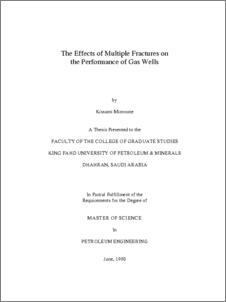# The Effect of multiple fractures on the performance of gas wells

Mimoune, Kissami (1990) The Effect of multiple fractures on the performance of gas wells. Masters thesis, King Fahd University of Petroleum and Minerals.Preview
PDF
3387Kb

-

## English Abstract

A single phase two dimensional mathematical model was used to predict the performance of a gas well intersected by two perpendicular vertical fractures. The fractures half lengths (X and Y ) were assumed to extend equally on both sides of the wellbore. The fractures were assumed to have either infinite or finite conductivities. The analysis of the simulated frawdown tests at constant flow rate showed that in the transient flow behavior of a gas well intersected by finite conductivities (F , <500) perpendicular fractures exhibit neither bilinear flow behavior nor formation linear flow behavior when Y /X is greater than zero. Instead it showed that the log-log plot of p versus t presented slopes higher than the characteristic slopes of the bilinear and linear flow periods. On the other hand when the fractures conductivities were equal to or greater than 500, the formation linear flow period was observed. The square-root method was used to determine the fracture half length from the formation linear flow period and found to give a fracture half length that is equal to the sum of the fracture half lengths conductivities up to 0.2 , the pressure drops, p , calculated for the cases where y /x are greater than zero were lower than those for single fracture (y /x = 0.0). But, as the fracture conductivity increased the calculated pressure drops were found to increase to values higher than those for the single fracture cases after some time. This time was found to be a function of fracture conductivity where it decreased as the fracture conductivity increased. The results when plotted as a function of p versus logt for both infinite and finite conductivities perpendicular vertical fractures gave, for large t , a 1.151-slope straight line. Hence, semilogarithmic pressure analysis methods can be applied.

Item Type: Thesis (Masters) June 1990 Completion Petroleum College Of Engineering Sciences > Petroleum Engineering Dept Mimoune, Kissami Al-Hashim, Hasan S. Al-Yousef, Hasan Yousef and Aggour, Mohammad A. 10169 KFUPM ePrints Admin 22 Jun 2008 16:58 25 Apr 2011 10:24

Repository Staff Only: item control page